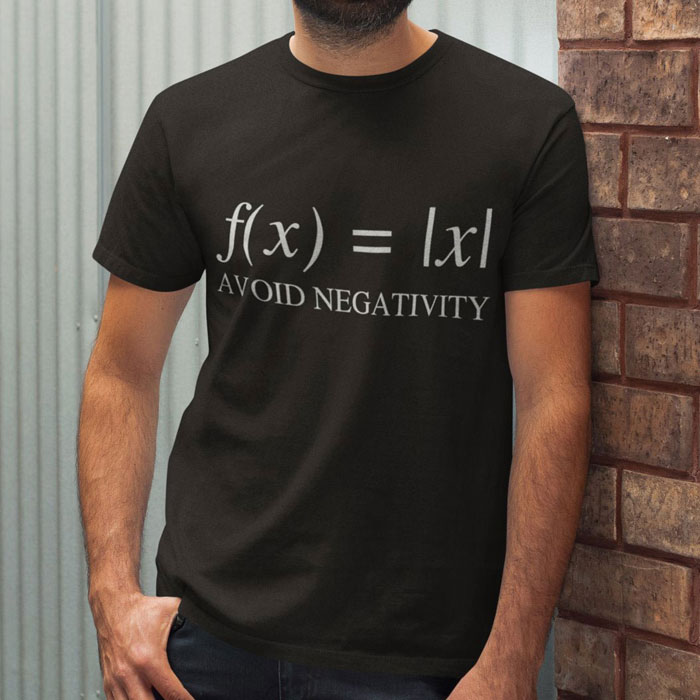A# absolute valueAbsolutely!

Absolute value is the value of a number without regard to its sign. The absolute value, or modulus, of a real number, r, is just the distance of the number from zero measured along the real number line, and is denoted |r | (read "mod r "). Being a distance, it can't be negative; so, for example, |3| = |-3| = 3.

The same idea applies to the absolute value of a complex number a + ib, except that, in this case, the complex number is represented by a point on an Argand diagram. The absolute value, |a + ib|, is the length of the line from the origin to the given point, and is equal to √(a2 + b2).